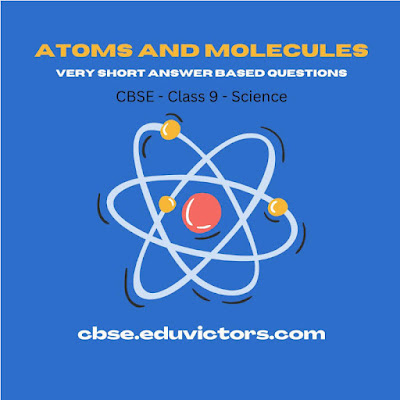# CBSE Class 9 - Chemistry  - Atoms and Molecules - Very Short Answer Based Questions - Answers

Q1. What is the chemical symbol of lead and mercury?

Q2. Monika finds that all samples of pure water have Hydrogen and Oxygen in ratio of 1:8 by mass. Which law does the statement agree to?

Q3. 10g of silver nitrate solution is added to 10g of sodium chloride solution. What change in mass do you expect after the reaction and why?

Q4. What is the difference between O, O₂ and O₃?

Q5. Define molecule.

Q6. Categorise the following molecules as monoatomic, diatomic, triatomic, tetraatomic, Pentaatomic and polyatomic.

CH₄, H₂O, He, S₈, N₂, NH₃

Q7. What is the atomic radius of a Hydrogen atom in metres?

Q8. Define atomic mass unit.

Q9. Give an example of bilavent cation.

Q10. Calculate the ratio by mass of atoms present in — a molecule of carbon dioxide.

(Given C = 12u, O=16u)

Q11. How many atoms are present in a PO₄²⁻ ion?

Q12. Write chemical formula of Lead Carbonate.

Q13. What is the chemical name of K₂SO₄ ?

Q14. What is the chemical name of Quicklime?

Q15. Calculate the molecular mass of Ethyne C₂H₂.

Mercury - Hg

2. Law of constant proportion.

3. Since Law of conservation of mass hold good, there is no change in mass will take place.

4. O - Oxygen atom,

O₂ - Oxygen gas molecule (diatomic) and

O₃ - Ozone gas molecule (triatomic)

5. The smallest unit of a substance which can exist independently is called molecule.

6. Monoatomic: He

Diatomic: N₂

Triatomic: H₂O

Tetraatomic: NH₃

Pentaatomic: CH₄

Polyatomic: S₈

7. 10⁻¹⁰ m

8. Atomic mass unit is equal to 1/12th the mass of one atom of carbon-12.

9. Mg²⁺

10. The ratio by mass of constituting elements in carbon dioxide is:

CO₂ = 12:32 = 3:8

11. 1 P + 4 O atoms = 5 atoms

12. PbCO₃

13. Pottassium Sulphate

14. Calcium Oxide CaO

15. C₂H₂.

Atomic mass of C = 12u

Atomic mass of H = 1u

Molecular mass of C₂H₂ = 2×12u + 2×1u

= 24u + 2u

= 26u